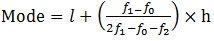Guru

# During the medical check-up of 35 students of a class, their weights were recorded as follows:Draw a less than type ogive for the given data. Hence obtain the median weight from the graph and verify the result by using the formula. Q.2

• 0

How i solve this problem from of exercise 14.4 of statistics chapter of question no.2 of ncert class 10th math book, give me the best solution for this question give me the best way for this question  During the medical check-up of 35 students of a class, their weights were recorded as follows:Draw a less than type ogive for the given data. Hence obtain the median weight from the graph and verify the result by using the formula.

Share

1. The class 46 – 48 has the maximum frequency, therefore, this is modal class

Here, = 46, h = 2, f1= 14, f0= 5 and f2 = 4

The mode formula is given as:

Now, Mode == 46 + 0.95 = 46.95

Thus, mode is verified.

• 0